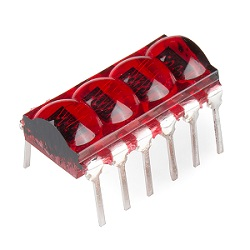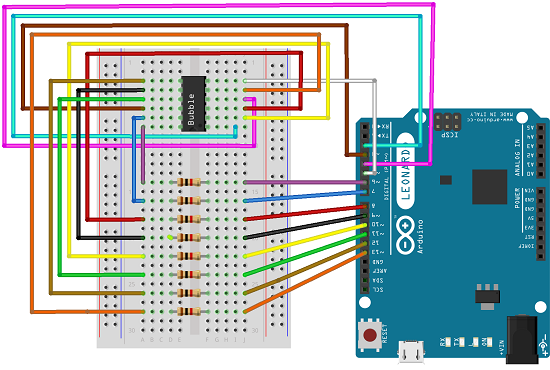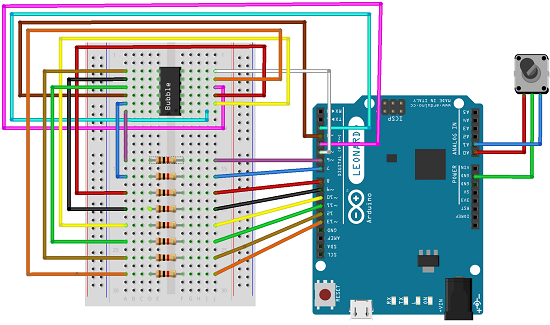# Bubble Display

## Introduction

In this project you will be able to use a 4 digit, 7 segment bubble display to count numbers.

## You Will Need

• 8x 1K Ohm Resistors
• Lots of jumper wires
• The Library for the sketch, here
• A sparkfun Bubble display. You can get one here

The bubble display looks like this## Making the circuit

Here is a sketch that shows you how to make the circuit## Coding

This sketch will count from 1 to 9999

```/*  March 6, 2014  Spark Fun Electronics  Nathan Seidle  Updates by Joel Bartlett    This code is originally based Dean Reading's Library deanreading@hotmail.com  http://arduino.cc/playground/Main/SevenSegmentLibrary  He didn't have a license on it so I hope he doesn't mind me   making it public domain:   This code is public domain but you buy me a beer if you use this   and we meet someday (Beerware license).    This sketch provides a simple counter example for the   HP Bubble display from SparkFun.  https://www.sparkfun.com/products/12710    Pinout for HP Bubble Display:  1:  Cathode 1  2:  Anode E  3:  Anode C  4:  Cathode 3  5:  Anode dp  6:  Cathode 4  7:  Anode G  8:  Anode D  9:  Anode F  10: Cathode 2  11: Anode B  12: Anode A  */ #include "SevSeg.h" //Create an instance of the object. SevSeg myDisplay; //Create global variables unsigned long timer; int deciSecond = 0; void setup() {   int displayType = COMMON_CATHODE;   //Your display is either common cathode or common anode        //This pinout is for a bubble dispaly        //Declare what pins are connected to the GND pins (cathodes)        int digit1 = 2; //Pin 1        int digit2 = 3; //Pin 10        int digit3 = 4; //Pin 4        int digit4 = 5; //Pin 6                //Declare what pins are connected to the segments (anodes)        int segA = 6; //Pin 12        int segB = 7; //Pin 11        int segC = 8; //Pin 3        int segD = 9; //Pin 8        int segE = 10; //Pin 2        int segF = 11; //Pin 9        int segG = 12; //Pin 7        int segDP= 13; //Pin 5       int numberOfDigits = 4; //Do you have a 1, 2 or 4 digit display?   myDisplay.Begin(displayType, numberOfDigits, digit1, digit2, digit3,    digit4, segA, segB, segC, segD, segE, segF, segG, segDP);      myDisplay.SetBrightness(100); //Set the display to 100% brightness level   timer = millis(); } void loop() {   //Example ways of displaying a decimal number   char tempString; //Used for sprintf   sprintf(tempString, "%4d", deciSecond); //Convert deciSecond into a string that is right adjusted   //sprintf(tempString, "%d", deciSecond); //Convert deciSecond into a string that is left adjusted   //sprintf(tempString, "%04d", deciSecond); //Convert deciSecond into a string with leading zeros   //sprintf(tempString, "%4d", deciSecond * -1); //Shows a negative sign infront of right adjusted number   //sprintf(tempString, "%4X", deciSecond); //Count in HEX, right adjusted   //Produce an output on the display   myDisplay.DisplayString(tempString, 0); //(numberToDisplay, decimal point location)   //Other examples   //myDisplay.DisplayString(tempString, 0); //Display string, no decimal point   //myDisplay.DisplayString("-23b", 3); //Display string, decimal point in third position   //Check if 10ms has elapsed   if (millis() - timer >= 100)   {     timer = millis();     deciSecond++;   }   delay(5); }```

This code is from the library, if you want to use the stock code make sure you change the pins to what I have here

## Introduction

You can add a rotary encoder to the circuit so that it counts up and down as you turn the rotary encoder.

## You Will Need

• 8x 1K Ohm Resistors
• Lots of jumper wires
• The Library for the sketch, here
• A sparkfun Bubble display. You can get one here
• A rotary enoder

## Making the circuit

Below is a sketch which shows you how to make the circuit.## Coding

Here is the sketch,

```/*  Pinout for HP Bubble Display:  1:  Cathode 1  2:  Anode E  3:  Anode C  4:  Cathode 3  5:  Anode dp  6:  Cathode 4  7:  Anode G  8:  Anode D  9:  Anode F  10: Cathode 2  11: Anode B  12: Anode A  */ #include "SevSeg.h" int changeamnt = 1; //Create an instance of the object. unsigned long currentTime; unsigned long lastTime; const int pinA = A0; const int pinB = A1; boolean encA; boolean encB; boolean lastA =false; SevSeg myDisplay; unsigned long timer; int reading = 0; int lowest = 0; int highest = 9999; int deciSecond = 0; //Create global variables void setup() {   pinMode(pinA, INPUT_PULLUP);   pinMode(pinB, INPUT_PULLUP);   currentTime = millis();   lastTime = currentTime;   int displayType = COMMON_CATHODE; //Your display is either common cathode or common anode        //This pinout is for a bubble dispaly        //Declare what pins are connected to the GND pins (cathodes)        int digit1 = 2; //Pin 1        int digit2 = 3; //Pin 10        int digit3 = 4; //Pin 4        int digit4 = 5; //Pin 6                //Declare what pins are connected to the segments (anodes)        int segA = 6; //Pin 12        int segB = 7; //Pin 11        int segC = 8; //Pin 3        int segD = 9; //Pin 8        int segE = 10; //Pin 2        int segF = 11; //Pin 9        int segG = 12; //Pin 7        int segDP= 13; //Pin 5                       int numberOfDigits = 4; //Do you have a 1, 2 or 4 digit display?   myDisplay.Begin(displayType, numberOfDigits, digit1, digit2, digit3,  digit4, segA, segB, segC, segD, segE, segF, segG, segDP);      myDisplay.SetBrightness(100); //Set the display to 100% brightness level   timer = millis();   Serial.begin(9600); } void loop() {   currentTime = millis();      if(currentTime >= (lastTime + 5))   {     Serial.println(reading);     char tempString;     sprintf(tempString, "%4d", reading);     myDisplay.DisplayString(tempString, 0);     encA = digitalRead(pinA);     encB = digitalRead(pinB);          if ((!encA) && (lastA))      {        // check if B is high       if (encB)        {          // clockwise          if (reading + changeamnt <= highest)          {            reading = reading + changeamnt;           }        }        else        {          // anti-clockwise          if (reading - changeamnt >= lowest)          {            reading = reading - changeamnt;           }        }   //Produce an output on the display   myDisplay.DisplayString(tempString, 0);  //(numberToDisplay, decimal point location)   //Other examples   //myDisplay.DisplayString(tempString, 0); //Display string, no decimal point   //myDisplay.DisplayString("-23b", 3); //Display string, decimal point in third position   //Check if 10ms has elapsed   if (millis() - timer >= 100)   {     timer = millis();     deciSecond++;   }   delay(5);      }   } } ```

This sketch will do what I described above.